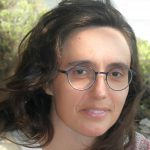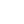Select Page

# Valuation Theory and the OM Algorithm

From January 15, 2024
to January 19, 2024

Dates: From January 15 to 19, 2024

Location: UPC Facultat de Matemàtiques i Estadística (Sala: S05 FME) | Centre de Recerca Matemàtica (Auditorium)

Format: onsite

Schedule:

• Monday 15: from 16h to18h (CRM AUDITORIUM)
• Tuesday 16, Wednesday 17 and Thursday 18: from 9h to 11h / from 11'30h to 13'30h (FME-UPC)
• Friday 19: from 10h to 12h (FME-UPC)

* Registration is free but mandatory

Registration deadline 10 / 01 / 2024

Introduction

Valuation theory offers a common framework for relevant questions in Algebraic Number Theory and Algebraic Geometry.

A fundamental question in valuation theory is the extension problem: given a valued field (K, v) and a monic irreducible polynomial f ∈ K[x], compute all extensions of v to the finite simple extension L = K[x]/(f).

If K is a number field and v is the valuation associated to a prime ideal p in the ring of integers of K, then the extension problem amounts to the computation of the prime ideal decomposition of p in the ring of integers of L.

If C is a curve over an algebraically closed field k of zero characteristic, L = k(C) is its function field and K = k((p)) with p an indeterminate, then computing the extensions of v is equivalent to the decomposition of C into branches at the origin and to parameterize these branches using Puiseux series.

The aim of this course is to analyze a computational resolution of the extension problem given by the OM-algorithm, named after Ore, Mac Lane, Okutsu and Montes. This algorithm uses a routine of polynomial factorization over finite extensions of the residue class field k of the initial valuation v as an essential ingredient. As a consequence, it is specially efficient when k is a finite field, as is the case for number fields and curves over finite fields.

Recent advances in the Mac Lane–Vaquié theory of key polynomials of valuations over the ring K[x] will be reviewed. These tools will be used to reformulate the algorithm in a more elegant and comprehensive way. Some ideas to extend the algorithm beyond the classical rank-one discrete case will be presented too.

Organisers

Maria Alberich (Universitat Politècnica de Catalunya)

Jordi Guàrdia (Universitat Politècnica de Catalunya – CRM)

Enric Nart (Universtat Autònoma de Barcelona)

Joaquim Roé (Universtat Autònoma de Barcelona)

SPEAKERS#### Maria Alberich

##### Universitat Politècnica de Catalunya

Her doctoral thesis laid the groundwork for the modern theory of plane Cremona maps. With expertise in Singularity Theory from an Algebraic Geometry perspective, she focuses on the local geometry of singularities, emphasizing topological and analytical invariants such as multiplier ideals and the Bernstein-Sato polynomial, along with a keen interest in valuation theory. Additionally, she has contributed to mathematical modeling in robotics.#### Jordi Guàrdia

##### Universitat Politècnica de Catalunya

Jordi Guàrdia is specialized in Number Theory and affiliated with the Number Theory Seminar of Barcelona and the Research Group in Number Theory at UPC. He received the Jaume Vicens Vives distinction for coordinating a project in 2022, and previously, the UPC Quality in University Teaching Award. In 2013, he was honored for his work on the ‘Germanic Roots of Contemporary Mathematics.’#### Enric Nart

##### Universitat Autònoma de Barcelona

Enric Nart is a member of the Number Theory Seminar. His research projects have focused on areas such as Arithmetic Algebraic Geometry, Combinatorial Methods in Arithmetic Geometry, and the applications of arithmetic geometry in cryptography, having over 60 publications on these fields.#### Sebastian Pauli

##### University of North Carolina Greensboro

His research is in computational number theory. He is particularly interested in algorithms for local fields and computational class field theory while investigating the distributions of the zeros of the (fractional) derivatives of the Riemann Zeta function.##### Université Lille

Adrien Poteaux completed his Ph.D. at the University of Limoges, under the guidance of Moulay Barkatou and Marc Rybowicz. His doctoral thesis, titled “Calcul de développements de Puiseux et application au calcul du groupe de monodromie d’une courbe algébrique plane,” was defended on October 15, 2008. You can access the thesis and presentation slides for more information.#### Joaquim Roé

##### Universitat Autònoma de Barcelona

His main research interests are multivariate interpolation, singularities, and valuative properties of algebraic curves. He contributed to the study of the Nagata conjecture and the Segre-Harbourne-Gimigliano-Hirschowitz conjecture, of degenerations of singularities in low dimensions, the Hilbert scheme and Seshadri constants. Recently he has become interested in valuation spaces and nonarchimedean analytic geometry.#### Martin Weimann

##### Université de Caen-Normandie

Martin Weimann’s academic journey includes a Ph.D. in Pure Mathematics from the University of Bordeaux I, around residue calculus in complex toric varieties under the supervision of Alain Yger. He works now at the University of Caen Normandie. His research projects focus on areas related to effective algebraic geometry, factorization of polynomials over global and local fields, or computational aspects of algebraic curves.

## Sessions:

### 1. Historical background. (2 hours: Alberich & Guàrdia)

• Curve desingularisation (Newton, Puiseux).
• Prime ideal decomposition in number fields (Kummer, Dedekind, Ore).
• Valuation theory as a common framework (Hensel, Krull, Mac Lane).

### 2-3. Valuation Theory. (4 hours: Weimann & Roé)

• Absolute values and completion.
• Extension of valuations.
• The fundamental inequality.
• Abhyankar’s inequality.
• Henselian valued fields.

### 4-5. Mac Lane-Vaquié theory. (4 hours: Nart & Alberich)

• Graded algebra of a valuation.
• Mac Lane-Vaquié key polynomials.
• Residual polynomial operators.
• Augmentation of valuations.
• Mac Lane-Vaquié chains.
• Characterisation of defect.
• Abstract key polynomials and Okutsu frames.

### 6-7-8. Computational aspects. (6 hours: Guàrdia, Pauli, Poteaux)

• Newton polygons.
• The OM algorithm.
• Approximate roots.
• Arithmetic and geometric applications.
• Complexity issues.
##### REFERENCES

 M. Alberich-Carramiñana, Alberto F. Boix, J. Fernández, J. Guàrdia, E. Nart, J. Roé, Of limit key polynomials, Illin. J. Math. 65, No.1 (2021), 201–229.
 M. Alberich-Carramiñana, J. Guàrdia, E. Nart, J. Roé, Valuative trees over valued fields, J. Alg. 614 (2023), 71–114.
 M. Alberich-Carramiñana, J. Guàrdia, E. Nart, J. Roé, Okutsu frames of irreducible polynomials over henselian fields, arXiv:2111.02811 [math.AC].
 M. Alberich-Carramiñana, J. Guàrdia, E. Nart, A. Poteaux, J. Roé, M. Weimann, Polynomial factorization over henselian fields, arXiv:2207.02139 [math.AC].
 J.-D. Bauch, Genus computation of global function fields, J. Symb. Comp. 66 (2015), 8–20.
 J.-D. Bauch, Computation of integral bases, J. Number Th. 165 (2016), 382—407.
 J.-D. Bauch, E. Nart, H. D. Stainsby, Complexity of OM factorizations of polynomials over local fields, LMS Journal of Computation and Mathematics 16 (2013), 139–171.
 E. Casas-Alvero, Singularities of plane curves, LMS Lecture Note Series 276 (2000), Cambridge University Press.
 J. Decaup, W. Mahboub, M. Spivakovsky, Abstract key polynomials and comparison theorems with the key polynomials of MacLane-Vaquié, Illinois J. Math. 62, Number 1-4 (2018), 253–270.
 O. Endler, Valuation Theory, Universitex, Springer-Verlag Berlin Heidelberg, 1972.
 A. J. Engler, A. Prestel, Valued fields, Springer, Berlin, 2005.
 J. Guàrdia, J. Montes, E. Nart, Okutsu invariants and Newton polygons, Acta Arith. 145 (2010), 83–108.
 J. Guàrdia, J. Montes, E. Nart, Higher Newton polygons in the computation of discriminants and prime ideal decomposition in number fields, J. Théor. Nombres Bordeaux 23 (2011), no. 3, 667–696.
 J. Guàrdia, J. Montes, E. Nart, Newton polygons of higher order in algebraic number theory, Trans. Amer. Math. Soc. 364 (2012), no. 1, 361–416.
 J. Guàrdia, J. Montes, E. Nart, A new computational approach to ideal theory in number fields, Found. Comput. Math. 13 (2013), 729–762.
 J. Guàrdia, J. Montes, E. Nart, Higher Newton polygons and integral bases, Journal of Number Theory 147 (2015), 549–589.
 J. Guàrdia, E. Nart, S. Pauli, Single-factor lifting and factorization of polynomials over local fields, Journal of Symbolic Computation 47 (2012), 1318–1346.
 J. Guàrdia, E. Nart, Genetics of polynomials over local fields, in Arithmetic, geometry, and coding theory, Contemp. Math. vol. 637 (2015), 207-241.
 F.J. Herrera Govantes, M.A. Olalla Acosta, M. Spivakovsky, Valuations in algebraic field extensions, Journal of Algebra 312 (2007), no. 2, 1033–1074.
 F.J. Herrera Govantes, W. Mahboub, M.A. Olalla Acosta, M. Spivakovsky, Key polynomials for simple extensions of valued fields, preprint, arXiv:1406.0657v4 [math.AG], 2018.
 F.-V. Kuhlmann, Valuation theory, book in preparation. Preliminary version of several chapters available at http://math.usask.ca/~fvk/Fvkbook.htm
 F.-V. Kuhlmann, Value groups, residue fields, and bad places of rational function fields, Trans. Amer. Math. Soc. 356 (2004), no. 11, 4559–4660.
 F.-V. Kuhlmann, The defect, in: Commutative Algebra – Noetherian and non-Noetherian perspectives, Marco Fontana, Salah-Eddine Kabbaj, Bruce Olberding and Irena Swanson (eds.), Springer 2011.
 W. Mahboub, Key polynomials, Journal of Pure and Applied Algebra 217 (2013), no. 6, 989–1006.
 S. MacLane, A construction for absolute values in polynomial rings, Trans. Amer. Math. Soc. 40 (1936), pp. 363–395.
 S. MacLane, A construction for prime ideals as absolute values of an algebraic field, Duke Mathematical Journal 2 (1936), pp. 492–510.
 J. Montes, Polígonos de Newton de orden superior y aplicaciones aritméticas, PhD Thesis, Universitat de Barcelona, 1999.
 N. Moraes de Oliveira, E. Nart, Defectless polynomials over henselian fields and inductive valuations, J. Algebra, 541 (2020), 270–307.
 N. Moraes de Oliveira, E. Nart, Computation of residual polynomial operators of inductive valuations, JPAA 225-9 (2021), 106668.
 E. Nart, Local computation of differents and discriminants, Math. Comp. 83 (2014), 1513–1534.
 E. Nart, Key polynomials over valued fields, Publ. Mat. 64 (2020), 195–232.
 E. Nart, MacLane-Vaquié chains of valuations on a polynomial ring, Pacific J. Math. 311-1 (2021), 165–195.
 J. Novacoski, M. Spivakovsky, Key polynomials and pseudo-convergent sequences, J. Algebra 495 (2018), 199–219.
 J. Novacoski, Key polynomials and minimal pairs, J. Algebra 523 (2019), 1–14.
 J. Novacoski, On MacLane–Vaquié key polynomials, JPAA 225 (2021), 106644.
 K. Okutsu, Construction of integral basis I, II, Proc. Japan Acad. Ser. A 58 (1982), 47–49, 87–89.
 Ø. Ore, Zur Theorie der algebraischen K¨orper, Acta Math. 44 (1923), pp. 219–314.
 Ø. Ore, Newtonsche Polygone in der Theorie der algebraischen K¨orper, Math. Ann. 99 (1928), 84–117.
 P. Popescu-Pampu, Approximate roots, Fields Inst. Comm. 33 (2002), 1–37.
 A. Poteaux, M. Weimann, A quasi-linear irreducibility test in K[[x]][y], J. Comput. Comp. 31 (2022), no. 6, 1–52.
 A. Poteaux, M. Weimann, Local polynomial factorisation: improving the Montes algorithm, Proceedings of the 2022 ACM on International Symposium on Symbolic and Algebraic Computation ISSAC’22 (2022), 149–158.
 H. D. Stainsby, Triangular bases of integral closures, J. Symb. Comp. 87 (2018) 140–175.
 M. Vaquié, Famille admise associée à une valuation de K[x], Singularités Franco-Japonaises, Séminaires et Congrès 10, SMF, Paris (2005), Actes du colloque franco-japonais, juillet 2002, édité par Jean-Paul Brasselet et Tatsuo Suwa, 391–428.
 M. Vaquié, Extension d’une valuation, Trans. Amer. Math. Soc. 359 (2007), no. 7, 3439–3481.
 M. Vaquié, Famille essential de valuations et défaut d’une extension, Journal of Algebra 311 (2007), no. 2, 859–876.
 M. Vaquié, Extensions de valuation et polygone de Newton, Annales de l’Institute Fourier (Grenoble) 58 (2008), no. 7, 2503–2541.
 M. Vaquié, Valuation augmentée et paire minimal, preprint 2020, hal-02565309.

LIST OF PARTICIPANTS

Name Institution
Caio Henrique Silva de Souza Federal University of São Carlos
Josep Sans Universitat Politècnica de Catalunya
Kamil Przybyszewski Universitat Politècnica de Catalunya
Javier Rivera Romeu Reed College
Elena Isasi Theus Universitat Politècnica de Catalunya
Javier Guillán Rial Centre de Recerca Matemàtica
Martín Alejandro Sombra Universitat de Barcelona
Xavier Povill Universitat Politècnica de CatalunyaFor inquiries about this event please contact the Scientific Events Coordinator Ms. Núria Hernández at nhernandez@crm.cat​​